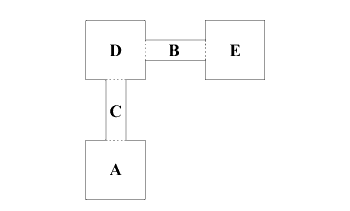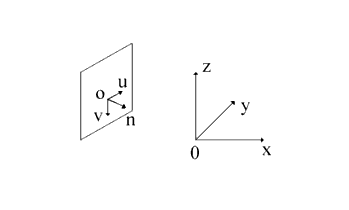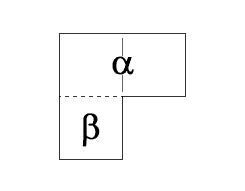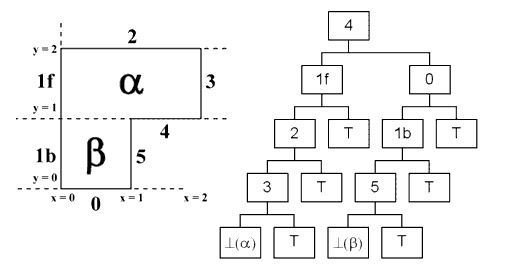# Through The Looking Glass

Through The Looking Glass

By Stuart Golodetz

What's happening in the next room? Stuart Golodetz has to find the door first!

In a previous article [ Golodetz08 ] , I talked about BSP trees and some of their uses for 3D games. One such use is in rendering a level: given an arbitrary position for the player camera, a BSP tree can be used to render the polygons in a scene in back-to-front order relative to the camera, with no need for a z-buffer. In practice, however, rendering a scene in this way will engender an unacceptably low frame-rate for all but the smallest levels (it's not a method that scales well).

One solution to this, as I mentioned briefly at the time, is to precalculate which empty leaves of a BSP tree can potentially see each other (recall that an empty leaf is called that because it represents an empty convex subspace of the world). For example, suppose there are five empty leaves ( A - E ): see Figure 1. If the player is standing in leaf A , and we know that leaf A cannot possibly see leaves B and E , then we need only render the polygons in leaves A , C and D . Needless to say, this makes rendering substantially faster.Figure 1

This precalculation process comes in two parts: first, we must determine the portals (or doorways) between adjacent leaves in the level (see the dotted lines in Figure 1). Having obtained these portals, we then calculate the visibility relation between them (i.e. which portals can see each other) in a manner which will be explained later. This portal visibility relation induces a related visibility relation between the leaves, which is what we're ultimately after.

Since the whole process is quite long and involved, I'll explain portal generation in this article, and save calculating the visibility relation for next time.

## Portal generation

For our purposes, a portal is a polygon which forms a directed link between adjacent (empty) leaves. Its normal will point in the direction of the link, i.e. away from the portal's from leaf and towards its to leaf. This means that portals are one-way , so we actually need two portals for each doorway, one pointing in each direction. For example, we might have a triangular portal from leaf A to leaf B (whose normal points towards B ), and another identical triangular portal (with reversed vertex winding order and opposite-facing normal) from leaf B to leaf A . (I will use counter-clockwise winding order for the purposes of this article, but it doesn't matter which you use as long as you're consistent.)

Generating portals is a three-step process:

1. Determine the set of undirected planes in which the portals could lie.
2. For each undirected plane:

## Determining the undirected plane set

Before we can generate any portals, we need to know the set of (undirected) planes in which they can potentially lie. (By 'undirected', I mean that we consider two otherwise identical planes which face in opposite directions to be the same, e.g. (1,0,0) . x - d = 0 is the same (in undirected plane terms) as (-1,0,0) . x + d = 0.) Intuitively, this seems quite simple: we just add the planes of all the polygons in our level to a set and we're done. In practice, though, we need to be careful to ensure that we don't get duplicate copies of the same plane. This is non-trivial because we're using floating-point values for our plane coefficients: for instance, as far as we're concerned, 1 x + 0 y - 2 z = 5 and 1.000001 x + 0 y - 1.99999 z = 4.99999 are basically the same plane, but to the computer they're different. What we need, then, is some way of clustering these very similar planes together.

A solution to this problem is to use a special ordering predicate for our plane set (see Listing 1). This compares two planes, lhs and rhs , and returns false if they are sufficiently similar. If not, it compares them lexicographically. The idea is that when we're inserting a new plane into a tree (using the normal procedure for set insertion), it can be 'captured'and rejected if it's too near one of the existing planes.

 ``` struct PlanePred { double m_angleTolerance, m_distTolerance; PlanePred(double angleTolerance, double distTolerance) : m_angleTolerance(fabs(angleTolerance)), m_distTolerance(fabs(distTolerance)) {} bool operator()(const Plane& lhs, const Plane& rhs) const { // If these planes are nearly the same (in // terms of normal direction and distance // value), then !(lhs < rhs) && !(rhs < lhs). double angle = acos( lhs.normal().dot(rhs.normal())); double dist = lhs.distance_value() - rhs.distance_value(); if(fabs(angle) < m_angleTolerance && fabs(dist) < m_distTolerance) return false; // Otherwise, compare the two planes // "lexicographically". const Vector3d& nL = lhs.normal(), nR = rhs.normal(); const double& aL = nL.x, bL = nL.y, cL = nL.z; const double& aR = nR.x, bR = nR.y, cR = nR.z; const double& dL = lhs.distance_value(), dR = rhs.distance_value(); return ((aL < aR) || (aL == aR && bL < bR) || (aL == aR && bL == bR && cL < cR) || (aL == aR && bL == bR && cL == cR && dL < dR)); } }; ``` Listing 1

Note that this is similar to the approach taken in [ Tampieri92 ] for grouping nearly coplanar polygons together. I've taken a simpler approach because only one plane from each nearly coplanar group is needed for the purposes of portal generation, so using a representative tree as per their article would be overkill here.

Using this predicate, then, the code to actually build the undirected plane set is quite simple (see Listing 2). Ignoring the templated stuff (which is necessary so that I can pass in either textured or non-textured polygons as input), all that's happening is as follows:

1. The undirected plane set is initialised with an instance of the predicate we created above. We pass in tolerance values to this for use in deciding when two planes are sufficiently similar.
2. We determine the undirected plane for each polygon in our level, and insert it into the set.
 ``` template typename PortalGenerator::PlaneList_Ptr PortalGenerator::find_unique_planes( const std::vector > >& polygons) { typedef Polygon Poly; typedef shared_ptr Poly_Ptr; typedef std::vector PolyVector; const double angleTolerance = 0.5 * PI / 180; // convert 0.5 degrees to radians const double distTolerance = 0.001; std::set planes( PlanePred(angleTolerance, distTolerance)); for(PolyVector:: const_iterator it=polygons.begin(), iend=polygons.end(); it!=iend; ++it) { Plane plane = make_plane( **it).to_undirected_form(); planes.insert(plane); } return PlaneList_Ptr( new PlaneList(planes.begin(), planes.end())); } ``` Listing 2

## Initial port generation

Having determined the planes in which the portals may lie, we now need to generate an initial portal on each of these planes. This should be a huge polygon which is large enough to span the entire level: the idea is that it's large enough to represent the entire plane for our purposes. We'll then clip it to the tree, which will give us the list of portals which lie on that plane (although as previously mentioned, we'll still need to make a reverse-facing copy of each of them).

Generating the initial portal itself is something that can be done in a variety of ways. The method I used (see Figure 2, which shows building an intiial portal on a plane) works as follows:

1. Generate an arbitrary unit vector, u, in the plane. To do this, we just calculate n x (0,0,1) (where n is the plane normal) and normalize the result, provided the angle between n and (0,0,1) isn't too small (since the cross product of two vectors which point in the same direction is the zero vector). If it is, we simply replace (0,0,1) by (1,0,0). Either way, we eventually end up with a vector which is perpendicular to n: it thus lies in the plane. (This gives us one axis of a coordinate system in the plane.)
2. Calculate u x n and normalize the result, to give another unit vector, v, in the plane which is perpendicular to u. (This gives us the other axis of the coordinate system.)
3. Project the world origin (0,0,0) onto the plane along the normal to give a point, o, in the plane. (This is the origin of the coordinate system.)
4. Generate a large square polygon on the planes with vertices at o + k(-u - v), o + k(u - v), o + k(-u + v) and o + k(u + v), for some arbitrarily large number k. (In practice, I chose k = 1000000: if you make it too large, you get small floating-point errors.)Figure 2

The code is shown in Listing 3, generating a large polygon on a plane.

 ``` double displacement_from_plane(const Vector3d& p, const Plane& plane) { const Vector3d& n = plane.normal(); double d = plane.distance_value(); // Note that this equation is valid precisely // because the plane normal is guaranteed to be // unit length by a datatype invariant of the // Plane class. return n.dot(p) - d; } Vector3d generate_arbitrary_coplanar_unit_vector( const Plane& plane) { const Vector3d& n = plane.normal(); Vector3d up(0,0,1); if(fabs(n.x) < EPSILON && fabs(n.y) < EPSILON) { // Special Case: n is too close to the // vertical, so n x up is roughly equal to // (0,0,0) // Use a different vector instead of up (any // different vector will do) and apply the // same method as in the else clause using the // new vector. return n.cross(Vector3d(1,0,0)).normalize(); } else { // The normalized cross product of n and up // satisfies the requirements of being // unit length and perpendicular to n (since // we dealt with the special case where n x up // is zero, in all other cases it must be // non-zero and we can normalize it to give us // a unit vector) return // n.cross(up).normalize(); } } template shared_ptr > make_universe_polygon(const Plane& plane, const AuxData& auxData) { typedef Polygon Poly; typedef shared_ptr Poly_Ptr; Vector3d origin(0,0,0); Vector3d centre = nearest_point_in_plane( origin, plane); Vector3d planarVecs; planarVecs = generate_arbitrary_coplanar _unit_vector(plane); planarVecs = planarVecs.cross( plane.normal()).normalize(); const double HALFSIDELENGTH = 1000000; for(int i=0; i<2; ++i) planarVecs[i] *= HALFSIDELENGTH; std::vector vertices; for(int i=0; i<4; ++i) vertices.push_back(centre); vertices -= planarVecs; vertices -= planarVecs; vertices -= planarVecs; vertices += planarVecs; vertices += planarVecs; vertices += planarVecs; vertices += planarVecs; vertices -= planarVecs; return Poly_Ptr(new Poly(vertices, auxData)); } Vector3d nearest_point_in_plane(const Vector3d& p, const Plane& plane) { /* Derivation of the algorithm: The nearest point in the plane is the point we get if we head from p towards the plane along the normal. */ double displacement = displacement_from_plane( p, plane); return p - displacement * plane.normal(); } ``` Listing 3

## Portal clipping

We now come to the most interesting bit of the portal generation algorithm: clipping the initial portal to the tree. This is done recursively, starting from the tree's root node (see Listing 4).

 ``` /** Clips the portal to the tree and returns a list of portal fragments which survive the clipping process. */ std::list PortalGenerator::clip_to_tree( const Portal_Ptr& portal, const BSPTree_Ptr& tree) { return clip_to_subtree(portal, tree->root()); } ``` Listing 4

At each stage of the recursive process, we clip a fragment of the initial portal (initially the whole thing) against a node of the tree. If the node is a branch node, we classify the portal against the node's split plane and take different actions depending on the result; if the node is a leaf, we discard the portal fragment if the leaf is solid, and (provided the leaf doesn't straddle the portal) note the leaf index in the portal fragment if the leaf is empty (this is done so that we can keep track of which empty leaves a valid portal connects). If an empty leaf does straddle a portal fragment (something which can easily happen: see Figure 3), we discard the fragment, since it isn't a doorway between two separate leaves.Figure 3

In Figure 3, the only valid portal is represented by the dotted line between a and b (in particular, the potential portal between a and itself is invalid)

The tricky bit is what to do for the various branch node cases, e.g. what action should we take if the portal fragment straddles the node's split plane? The cases where the portal is entirely behind or in front of a split plane are easy: we recurse down the appropriate side of the tree. For the straddling case, it suffices to split the portal across the plane, pass each half down the appropriate side of the tree, and then concatenate the results (see Listing 5).

 ``` /** Clips the portal to the subtree and returns a list of portal fragments which survive the clipping process. @param portal The portal to clip @param subtreeRoot The root of the subtree @param relativeToPortal The location of the subspace represented by the subtree relative to the portal (in front, behind, or straddling it) @return As stated */ std::list PortalGenerator::clip_to_subtree( const Portal_Ptr& portal, const BSPNode_Ptr& subtreeRoot, PlaneClassifier relativeToPortal) { if(subtreeRoot->is_leaf()) { const BSPLeaf *leaf = subtreeRoot->as_leaf(); if(leaf->is_solid()) return PortalList(); switch(relativeToPortal) { case CP_BACK: { portal->auxiliary_data().fromLeaf = leaf->leaf_index(); break; } case CP_FRONT: { portal->auxiliary_data().toLeaf = leaf->leaf_index(); break; } default: // CP_STRADDLE { // The portal fragment is in the middle of a // leaf (this is not an error, but we do // need to discard the portal fragment as // we'd otherwise have a portal linking a // leaf to itself). return PortalList(); } } PortalList ret; ret.push_back(portal); return ret; } else { const BSPBranch *branch = subtreeRoot->as_branch(); switch(classify_polygon_against_plane(*portal, *branch->splitter())) { case CP_BACK: { return clip_to_subtree(portal, branch->right(), relativeToPortal); } case CP_COPLANAR: { BSPNode_Ptr fromSubtree; BSPNode_Ptr toSubtree; if(branch->splitter()->normal().dot( portal->normal()) > 0) { fromSubtree = branch->right(); toSubtree = branch->left(); } else { fromSubtree = branch->left(); toSubtree = branch->right(); } PortalList fromPortals = clip_to_subtree( portal, fromSubtree, CP_BACK); PortalList ret; for(PortalListCIter it=fromPortals.begin(), iend=fromPortals.end(); it!=iend; ++it) { ret.splice(ret.end(), clip_to_subtree(*it, toSubtree, CP_FRONT)); } return ret; } case CP_FRONT: { return clip_to_subtree(portal, branch->left(), relativeToPortal); } case CP_STRADDLE: { // Note: The leaf links for the two half // polygons are inherited from the original // polygon here. SplitResults sr = split_polygon(*portal, *branch->splitter()); PortalList frontResult = clip_to_subtree(sr.front, branch->left(), relativeToPortal); PortalList backResult = clip_to_subtree(sr.back, branch->right(), relativeToPortal); PortalList ret; ret.splice(ret.end(), frontResult); ret.splice(ret.end(), backResult); return ret; } } } // The code will never actually get here, // because the switch above is exhaustive, // but the compiler still warns us because it // can't tell that. throw Exception("This should never happen"); } ``` Listing 5

The coplanar case is more intricate. First of all, we work out whether the portal is facing in the same direction as the plane or not by comparing the dot product of their normals to 0 (they're facing the same way if the dot product is positive). This determines which subtree of the current node is the from subtree (i.e. its root represents a convex subspace entirely behind the portal) and which is the to subtree (its root represents a convex subspace entirely in front of the portal). Having determined this, we pass the portal down one of the subtrees (the from subtree in my code) and clip it to the tree. We then clip the portal fragments which survived that clipping process to the other subtree (the to subtree in my code), and concatenate the results. Finally, we return the list of fragments which survived being clipped down both subtrees.

It is worth remarking on the role of the relativeToPortal function parameter in this process: it is there to indicate whether the subspace represented by the current node is in front of, behind, or straddling the portal. It is CP_STRADDLE at the start of the process (since the entire world space certainly straddles any portal), and only becomes either CP_FRONT or CP_BACK when the portal lies on a branch node's split plane (i.e. in the coplanar case we've just been discussing). At this point, we use relativeToPortal = CP_BACK for the from subtree (since that's entirely behind the portal) and relativeToPortal = CP_FRONT for the to subtree (since that's entirely in front of the portal). This allows us to correctly handle what happens to the portal when it ends up in a leaf.

## Bringing things together

We've now seen how to find the undirected plane set, how to generate an initial portal on each plane, and how to clip that portal to the tree. All that remains is to show the top-level code which ties all of this together and makes the reverse-facing copies of each portal (see Listing 6).

 ``` template typename PortalGenerator::PortalList_Ptr PortalGenerator::generate_portals( const std::vector > >& polygons, const BSPTree_Ptr& tree) { PortalList_Ptr portals(new PortalList); PlaneList_Ptr planes = find_unique_planes(polygons); for(PlaneList::const_iterator it= planes->begin(), iend=planes->end(); it!=iend; ++it) { Portal_Ptr portal = make_initial_portal(*it); portals->splice(portals->end(), clip_to_tree(portal, tree)); } // Generate the opposite-facing portals. for(PortalList::iterator it=portals->begin(), iend=portals->end(); it!=iend; ++it) { Portal_Ptr portal = *it; // Construct the reverse portal. Portal_Ptr reversePortal( portal->flipped_winding()); const PortalInfo& portalInfo = portal->auxiliary_data(); reversePortal->auxiliary_data() = PortalInfo(portalInfo.toLeaf, portalInfo.fromLeaf); // Insert it after the existing portal in the // list. ++it; it = portals->insert(it, reversePortal); } return portals; } ``` Listing 6

The only new bit in this is the code which makes the reverse-facing portals. This is largely trivial: all that's necessary is to flip the portal winding and switch the from and to leaves in the portal's auxiliary information structure.

## Example

It would be remiss of me not to show an example of all this in action, so let's walk through a bit of the portal generation for the small L-shaped room in Figure 4. To follow along, it might be easier if you work it through on a piece of paper!Figure 4
• The undirected plane set (where plane ax + by + cz = d is represented by the quadruple (a,b,c,d)) is {(1,0,0,0), (1,0,0,1), (1,0,0,2), (0,1,0,0), (0,1,0,1), (0,1,0,2)}, i.e. x = 0, x = 1, x = 2, y = 0, y = 1 and y = 2.
• Portal P 1 on plane x = 0
• Straddles 4 → split into P 1f and P 1b and recurse down each side
• P 1f is on the plane of 1f and same facing → the back subtree is the from subtree and the front subtree is the to subtree; pass down from subtree first
• Solid leaf → discard portal
• P 1b straddles 0 → split into P 1bf and P 1bb and recurse down each side
• P 1bf is on the plane of 1b and same facing → from := back, to := front; pass down from subtree first
• Solid leaf → discard portal
• P 1bb is in a solid leaf → discard it
• Portal P4 on plane y = 1
• On the plane of 4 and same facing → from := back, to := front; pass down from subtree first
• In front of 0 → recurse down front subtree
• Straddles 1b → split into P 4f and P 4b and recurse down each side
• P 4f straddles 5 → split into P 4ff and P 4fb and recurse
• β is empty → becomes from leaf of P 4ff
• P 4fb is in a solid leaf → discard it
• P 4b is in a solid leaf → discard it
• Now pass surviving fragments (i.e. P 4ff ) down the to subtree of 4
• In front of 1f, then 2, then 3, so ends up in leaf α, which becomes its to leaf
• Portal P 5 on plane x = 1
• Straddles 4 → split into P 5f and P 5b and recurse down each side
• P 5f is in front of 1f → recurse down front subtree
• P 5f straddles 2, so split it into P 5ff and P 5fb and recurse
• P 5ff is in front of 3 → recurse down front subtree
• P 5fb ends up in a solid leaf → discard it
• P 5b straddles 0, so split it into P 5bf and P 5bb and recurse
• P 5bf is in front of 1b → recurse down front subtree
• P 5bf is on the plane of 5 and opposite facing → from := front, to := back; pass down from subtree first
• β is empty → becomes from leaf of P 5bf
• Now pass P 5bf down the to subtree
• Solid leaf → discard portal
• P 5bb ends up in the solid leaf behind 0 → discard it

I'll leave generating portals on the remaining planes as an exercise for the reader. You'll note that so far only one portal (portal P 4ff , which goes from b to a) has survived the clipping process. (This is in fact the only portal -other than its reverse-facing duplicate, which goes from a to b - in this small level.)

## Conclusion

In this article, we've seen how to generate portals for a level. Next time, I'll explain how to use these to generate a leaf-to-leaf visibility table for our level as a way of speeding up level-rendering.

## References

[Golodetz08] 'Divide and Conquer: Partition Trees and Their Uses', Overload #86 , August 2008.

[Simmons01] 'Advanced 3D BSP, PVS and CSG Techniques', Gary Simmons and Adam Hoult, Game Institute, 2001.

[Tampieri92] 'Grouping nearly coplanar polygons into coplanar sets', Filippo Tampieri and David Salesin. In Graphics Gems III (ed. David Kirk), Academic Press, San Diego, July 1992.